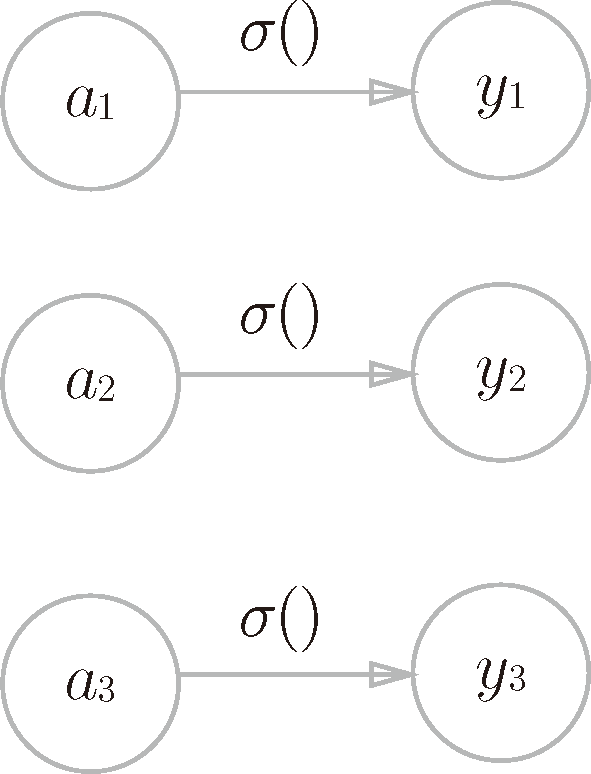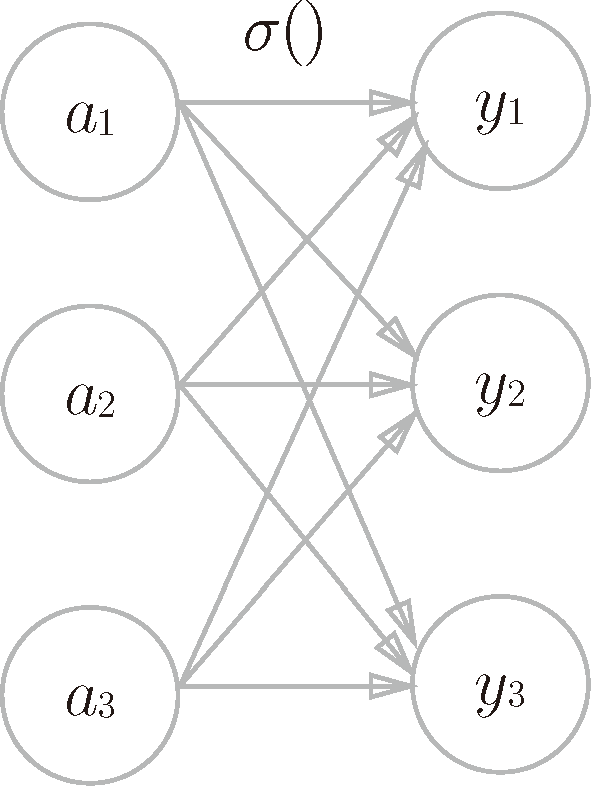# 深度学习入门：基于 Python 的理论与实现 (18)：神经网络 3.5&3.5.1(输出层的设计)

(恒等函数和 softmax 函数)$y_k=\frac{\exp(a_k)}{\sum^n_{i=1}\exp(a_i)}\quad\quad\quad\quad\quad(3.10)$

$\exp(x)$ 是表示 ${\rm e}^x$ 的指数函数（e 是纳皮尔常数 2.7182 …）。式（3.10）表示假设输出层共有 $n$ 个神经元，计算第 $k$ 个神经元的输出 $y_k$。如式（3.10）所示，softmax 函数的分子是输入信号 $a_k$ 的指数函数，分母是所有输入信号的指数函数的和。>>> a = np.array([0.3, 2.9, 4.0])
>>>
>>> exp_a = np.exp(a) #  指数函数
>>> print(exp_a)
[  1.34985881  18.17414537  54.59815003]
>>>
>>> sum_exp_a = np.sum(exp_a) #  指数函数的和
>>> print(sum_exp_a)
74.1221542102
>>>
>>> y = exp_a / sum_exp_a
>>> print(y)
[ 0.01821127  0.24519181  0.73659691]


def softmax(a):
exp_a = np.exp(a)
sum_exp_a = np.sum(exp_a)
y = exp_a / sum_exp_a

return y